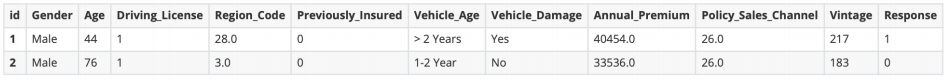# 健康保险的客户投保预测

## 一、题目简介

• 赛题背景:在一家保险公司里，通过保险公司的数据，预测一个人是否会对保险感兴趣。

• 数据示例:• 评价指标：AUC

## 二、赛题步骤

### 2.0 完整型判断

• 检测重复值 df_data.duplicated().sum()

• 检测列缺失值 df_data.isnull().sum(axis=0)

### 2.1 数据探索

• 探索离散值分布的常用代码。
 1 2 3 4 5 6  columns_list = ["Gender","Driving_License","Previously_Insured","Vehicle_Damage","Vehicle_Age","Response"] plt.figure(figsize=(10,5)) for id_,x in enumerate(columns_list): plt.subplot(2,3,id_+1) ## 这里注意我有6个属性，所以是2×3的子图 sns.countplot(data=df_data,x=x) plt.tight_layout() 

### 效果图• 探索连续值分布的常用代码
 1 2 3 4 5 6  plt.figure(figsize=(12,8)) x_list = ["Age","Region_Code","Annual_Premium","Policy_Sales_Channel","Vintage"] for id_,x in enumerate(x_list): plt.subplot(5,1,id_+1) ## 子图的大小和特征个数有关系 sns.distplot(df_data[x],kde=True) plt.tight_layout() 

### 效果图### 2.2 离散值编码

对于地区代码属性，其具有非常多的离散属性，我们可以使用目标编码进行编码，映射到一定的数值区间

  1 2 3 4 5 6 7 8 9 10 11 12 13 14 15 16 17 18 19 20 21 22 23 24 25 26 27 28 29 30  ## 标号编码 data["Gender"] = data["Gender"].map({"Male":1, "Female":0}) data["Vehicle_Age"] = data["Vehicle_Age"].map({"< 1 Year":0,"1-2 Year":1,"> 2 Years":2}) data["Vehicle_Damage"] = data["Vehicle_Damage"].map({"Yes":1,"No":0}) ## 目标编码，通过一个类可以更方便的实现 from sklearn.base import BaseEstimator, TransformerMixin class TargetEncoderTrain(BaseEstimator, TransformerMixin): def __init__(self, col, tar): self.col= col self.tar= tar self.discardOriginal_col = discardOriginal_col def fit(self, X): self.tar_mean = X[self.tar].mean() self.map = X.groupby(self.col)[self.tar].mean() return self def transform(self,X): col = self.col X[col] = X[col].map(self.map) X[col].fillna(self.tar_mean) return X et = TargetEncoderTrain("Region_Code","Response") train_data = pd.concat([x_train,y_train],axis=1) x_train[col] = et.fit_transform(train_data)[col] x_val[col] = et.transform(x_val)[col] 

### 2.3 特征之间相关性分析

 1 2 3  corr = df_data.corr() plt.figure(figsize=(16,10)) sns.heatmap(corr,annot=True,cmap="Purples") 

### 效果图### 2.4 寻找基础模型

  1 2 3 4 5 6 7 8 9 10 11 12 13 14 15 16 17 18 19 20 21 22 23 24 25 26 27 28 29 30 31 32 33 34  from sklearn.neighbors import KNeighborsClassifier from sklearn.linear_model import LogisticRegression from sklearn.tree import DecisionTreeClassifier from sklearn.ensemble import GradientBoostingClassifier from sklearn.ensemble import AdaBoostClassifier from sklearn.ensemble import RandomForestClassifier from sklearn.discriminant_analysis import LinearDiscriminantAnalysis from sklearn.naive_bayes import GaussianNB from sklearn.discriminant_analysis import QuadraticDiscriminantAnalysis from xgboost import XGBClassifier from lightgbm import LGBMClassifier from sklearn.metrics import roc_auc_score clf_list = { "KNN":KNeighborsClassifier(), "CART":DecisionTreeClassifier(), "LDA":LinearDiscriminantAnalysis(), "QDA":QuadraticDiscriminantAnalysis(), "LR":LogisticRegression(), "Ga":GaussianNB(), "GBDT":GradientBoostingClassifier(), "Adaboost":AdaBoostClassifier(), "RF": RandomForestClassifier(), "XGboost": XGBClassifier(), "LGBM": LGBMClassifier(), } score_list = list() for name,clf in clf_list.items(): clf.fit(x_train,y_train) y1 = clf.predict_proba(x_val)[:,1].flatten() score = roc_auc_score(y_val,y1) print(name,score,end="\n\n") 

Tip

### 2.4 模型调参

  1 2 3 4 5 6 7 8 9 10 11 12 13 14 15 16 17 18 19 20 21 22 23 24 25 26 27 28 29 30 31 32 33 34 35 36 37  from hyperopt import fmin, tpe, hp, Trials, space_eval ## 定义参数空间 param_space = {'max_depth': hp.choice('max_depth', range(4, 9, 1)), 'reg_lambda': hp.uniform('reg_lambda', 0.0, 1.0), 'learning_rate' : hp.quniform('learning_rate', 0.1, 0.5, 0.1), 'n_estimators' : hp.choice('n_estimators', range(50, 250, 30)), 'num_leaves': hp.choice('num_leaves',[15, 31, 63, 127]), 'subsample': hp.choice('subsample',[0.6, 0.7, 0.8, 1.0]), 'colsample_bytree': hp.choice('colsample_bytree',[0.6, 0.7, 0.8, 1.0]),} ## 定义评判函数，调参机器认为返回值越小越好，所以返回1-AUC def objective_lgbm(params): x_train,x_val,y_train,y_val = train_test_split(train,labels,test_size=0.1,random_state=0,stratify=labels) x_train,x_val,y_train, = target_labels(x_train,x_val,y_train) clf = LGBMClassifier(**params) clf.fit(x_train,y_train) y1 = clf.predict_proba(x_val)[:,1].flatten() score = roc_auc_score(y_val,y1) return 1 - score ## 八股文代码 trials = Trials() best = fmin(fn=objective_lgbm, space=param_space, max_evals=10, ## 迭代的次数 rstate=np.random.RandomState(0), algo=tpe.suggest, trials=trials ) ## 查看最佳代码 best_params = space_eval(param_space, best) print("最佳参数:",best_params) ## 之后直接创建最佳模型 clf = LGBMClassifier(**best_params)